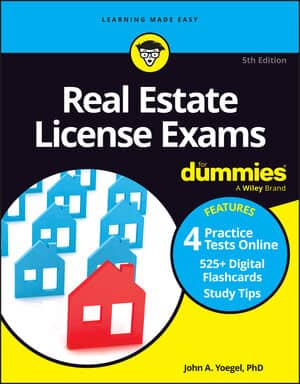##### Real Estate License Exams For DummiesUsing percentages is one type of math problem on the Real Estate License Exam. Commissions usually are figured on a percentage basis. Shared ownership of a property may be on a percentage basis. Vacancy rates usually are expressed as a percent. You’ll work with percents to figure out the selling price of a property. Property tax calculations may also involve percentages.

These basics can be applied to any problems asking you to use or calculate percentages. Some calculators have percentage keys that you can use. Feel free to use them, if you know how, or ignore them.

A percent — which is expressed as a number like 4 percent, 8 percent, 7.5 percent, or 0.8 percent — essentially describes what part of a hundred you’re talking about. The word “percent” comes from the Latin word centum, meaning a hundred. So 4 percent means four parts per hundred of whatever you’re taking about.

If 4 percent of the eggs in a truckload are bad, then four out of every hundred eggs are bad. And if you’re allowed to build on 4 percent of your land area, it means you can cover 4 square feet out of every 100 square feet with a building. The calculations you do with percentages usually are most often multiplication and occasionally or division, depending on the problem.

You have two choices for turning a percentage into a number you can work with. You can either divide the percent number by 100 or move the decimal point two places to the left.

Say, for example, that you have to use 7 percent in a problem. If you divide 7 by 100, you get a number you can use in a calculator or by hand.

7 ÷100 = 0.07

That isn’t 0.07 percent. It’s simply 0.07, which is the same as 7 percent.

Another way to remember is that if a decimal point doesn’t actually appear in the number, then it’s assumed to be at the end. From there (the right side of a whole number), move the decimal to the left two spaces, even if you have to add one or more zeroes. Seven percent becomes 0.07. Check out a few more of these:

800%→8

80%→0.80

8%→0.08

.8%→0.008

.08%→0.0008

8.5%→0.085

Note: You probably won’t be working too much with small percentages, such as 0.08 percent or 0.8 percent, either on an exam or in real life.

Don’t forget the reverse of this concept so you can solve a problem like this.

You make a \$20,000 commission on the sale of a \$400,000 house. What is your commission rate? Keep in mind that the word rate usually means percent. The calculation is

\$20,000 ÷\$400,000 = 0.05

Move the decimal point two places to the right to get the answer of 5 percent. Remember, to get from a decimal to a percentage, move the decimal two places to the right, or multiply by a hundred.

One other thing you need to know how to do is to convert a fraction to a decimal and, in turn, a percentage. Don’t worry. If you don’t remember your sixth-grade math class, this step isn’t too difficult. Just divide the upper number (numerator) by the lower number (denominator). Doing so gives you a decimal. Now move the decimal point two places to the right and you have a percentage.

Check out the following examples:

1/2 = 1 ÷2 = 0.5 = 50 percent

3/8 = 3 ÷8 = 0.375 = 37.5 percent

9/16 = 9 ÷16 = 0.5625 = 56.25 percent